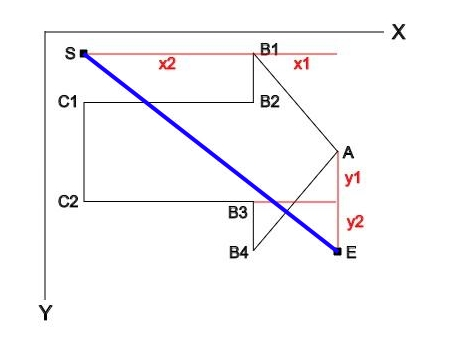# 名詞定義input：

• 起始點 `S` 座標為 (sx, sy)
• 終止點 `E` 座標為 (ex, ey)
• 比例參數：`rx1``rx2``ry1``ry2`
• 角度：`d`
• 在上圖中 `d = 0`。箭頭向下是 `d=90`

• `w`：abs(ex - sx)
• `h`：abs(ey - sy)
• `x2`：w * rx2 / (rx1 + rx2)
• `y2`：h * ry2 / (ry1 + ry2) / 2

• `A`：(ex, (sy + ey) / 2)
• `B1`：(sx + x2, sy)
• `B2`：(sx + x2, sy + y2)
• `C1`：(sx, sy + y2)
• `C2`：(sx, ey - y2)
• `B3`：(sx + x2, ey - y2)
• `B1`：(sx + x2, ey)

# 實作

``````var points = [];
points.push([ex, (sy + ey) / 2]);   //A
points.push([sx + x2, sy]); //B1
points.push([sx + x2, sy + y2]);    //B2
points.push([sx, sy + y2]); //C1
points.push([sx, ey - y2]); //C2
points.push([sx + x2, ey - y2]);    //B3
points.push([sx + x2, ey]); //B4

canvas.beginPath();
canvas.moveTo(points, points);

for (var i = 0; i < points.length; i++) {
canvas.lineTo(points[i], points[i]);
}

canvas.fill();
canvas.closePath();
``````

## 旋轉

CanvasRenderingContext2D`rotate()`，但它的運算邏輯是對於原點作 rotate，所以假設要用上面那組 `points` 搭配 `rotate()` 畫出向右的箭頭：

``````var d = 180;
d = d * Math.PI / 180;  //rotate() 吃的是弳度，所以要轉換
canvas.rotate(d * Math.PI / 180);

canvas.beginPath();
//後略......
``````

1. 把箭頭中心移動到原點：`translate(-tx, -ty)`
2. 旋轉：`rotate(d)`
3. 把箭頭中心移動回原位：`translate(tx, ty)`

``````//這裡的 d 已經轉換成弳度了
var sinD = Math.sin(d),
cosD = Math.cos(d);

canvas.transform(
cosD, sinD,
-sinD, cosD,
tx * (1 - cosD)+ ty * sinD, ty * (1 - cosD) - tx * sinD
);

canvas.beginPath();
//中間一樣，略
canvas.closePath();

//把 transform matrix 設回正常的樣子以免妨礙後續操作
canvas.setTransform(1, 0, 0, 1, 0, 0);
``````

## 90 / 270 度的問題

`d` 為 90 / 270 時，會發現產生的箭頭形狀似乎不如預期。這是因為最終箭頭的 y 軸長度，其實是來自於 `w`。也就是說本來預期會出現一個細長的向下箭頭，實則出現的是一個粗短的向下箭頭。以此類推，在 `d` 落在 45～135 或是 225～315 區間時，要給另一組 `points` 才能接近預期。

``````if ((degree > 45 && degree < 135) || (degree > 225 && degree < 315)) {
//points[] 的內容值自己算...
d = d - 90;
} else {
//原本向右的 points
}

//後面都一樣
``````